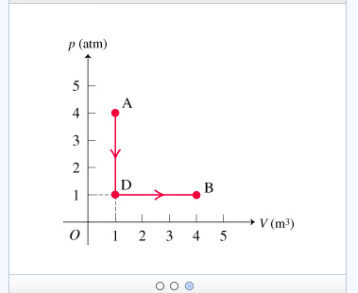# Problem: Isobaric, Isochoric, Isothermal, and Adiabatic Processes Learning Goal: To recognize various types of processes on pV diagrams and to understand the relationship between pV-diagram geometry and the quantities Q, W, and ΔU. The first law of thermodynamics is an expression of conservation of energy. This law states that changes in the internal energy of a system Δ U can be explained in terms of energy transfer into or out of the system in the form of heat Q and/or work W. In this problem, we will write the first law of thermodynamics as Δ U = Qin-Qout + Win-Wout. Here "in" means that energy is being transferred into the system, thereby raising its internal energy, and "out" means that energy is leaving the system, thereby reducing its internal energy.How are Q and W related during the isochoric part of the overall path from state A to state D? a) Both Q and W equal zero. b) Q provides energy input, while W equals zero. c) Q provides energy output, while W equals zero. d) Q provides energy input, while W provides energy output. e) Q provides energy output, while W provides energy input.

###### FREE Expert Solution

The first law of thermodynamics:

$\overline{){\mathbf{d}}{\mathbf{Q}}{\mathbf{=}}{\mathbf{d}}{\mathbf{U}}{\mathbf{+}}{\mathbf{d}}{\mathbf{W}}}$

dU is the internal energy, and dW is the work done by the system.

Thus, dW = PΔV

ΔV = 0 from A to D.

Implying that dW = 0

97% (199 ratings)###### Problem Details

Isobaric, Isochoric, Isothermal, and Adiabatic Processes

Learning Goal: To recognize various types of processes on pV diagrams and to understand the relationship between pV-diagram geometry and the quantities Q, W, and ΔU.

The first law of thermodynamics is an expression of conservation of energy. This law states that changes in the internal energy of a system Δ U can be explained in terms of energy transfer into or out of the system in the form of heat Q and/or work W. In this problem, we will write the first law of thermodynamics as Δ U = Qin-Qout + Win-Wout

Here "in" means that energy is being transferred into the system, thereby raising its internal energy, and "out" means that energy is leaving the system, thereby reducing its internal energy.How are Q and W related during the isochoric part of the overall path from state A to state D?

a) Both Q and W equal zero.

b) Q provides energy input, while W equals zero.

c) Q provides energy output, while W equals zero.

d) Q provides energy input, while W provides energy output.

e) Q provides energy output, while W provides energy input.